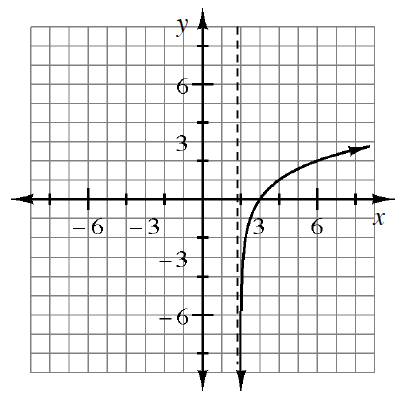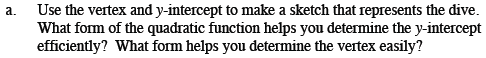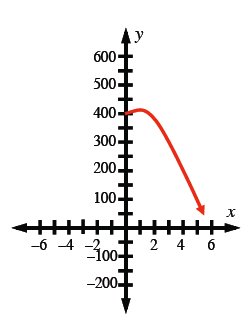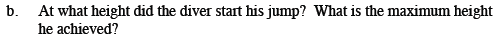Home > CCA2 > Chapter 8 > Lesson 8.2.2 > Problem8-95

8-95.
1. The La Quebrada Cliff Divers perform shows for the public by jumping into the sea off the cliffs at Acapulco, Mexico. The height (in feet) of a diver at a certain time (in seconds) is given by h = −16t2 +16t + 400. Homework Help ✎

1. Use the vertex and y-intercept to make a sketch that represents the dive. What form of the quadratic function helps you determine the y-intercept efficiently? What form helps you determine the vertex easily?

2. At what height did the diver start his jump? What is the maximum height he achieved?

2.What height is the diver when t = 0?

Complete the square to write the graphing form of the equation. This will indicate the vertex.

$h=-16(t^2-t+?)+400-?$

$h=-16\left(t^2-t+\frac{1}{4}\right)+400-\left(-16×\frac{1}{4}\right)$

Factor the polynomial in the first set of parentheses to get the equation into graphing form.The diver started at 400 feet. The diver reached a maximum height of 404 feet.